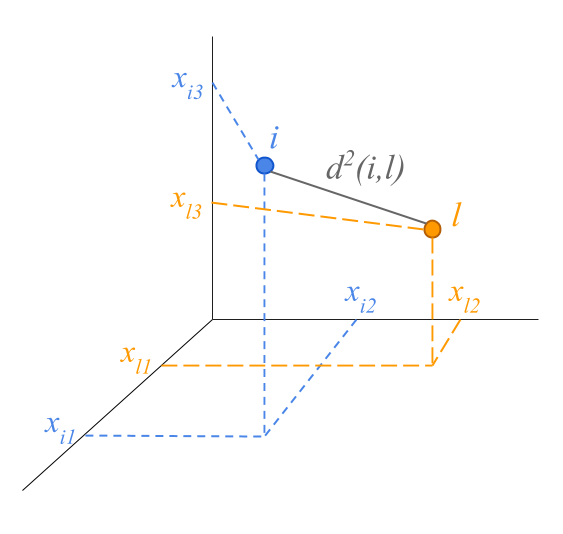5.2 Distances between points

The squared of the distance between two points can be calculated by the sum of the squared of the differences between coordinates:

$d^2(i,l) = (x_{i1} - x_{l1})^2 + (x_{i2} - x_{l2})^2 + (x_{i3} - x_{l3})^2$The distance formula can be extended to spaces with more than three dimensions.

$d^2(i,l) = (x_{i1} - x_{l1})^2 + (x_{i2} - x_{l2})^2 + \dots + (x_{ip} - x_{lp})^2$